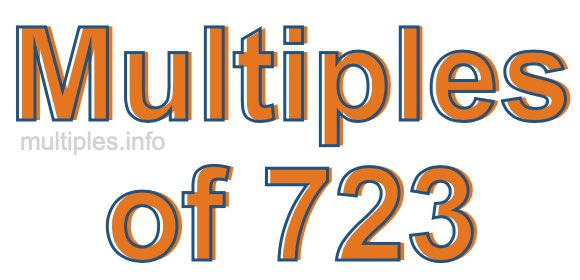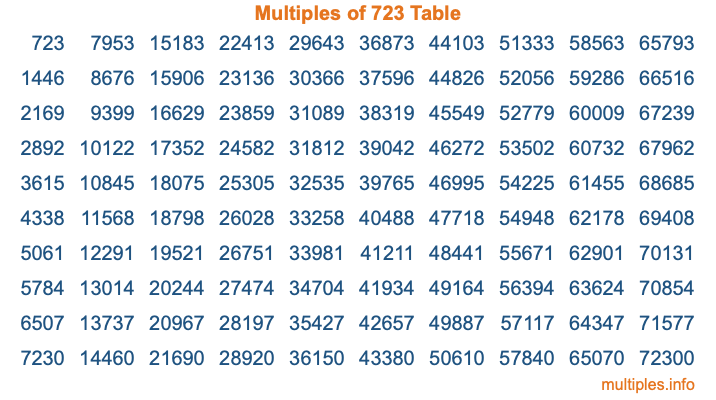Multiples of 723Welcome to the Multiples of 723 page. Here we will first teach you everything you will ever need to know about the multiples of 723, and then give you a study guide summary of everything we taught you to make sure you remember it all. Use this page to look up facts and learn information about the multiples of 723. This page will make you a multiples of seven hundred twenty-three expert!

Definition of Multiples of 723
Multiples of 723 are all the numbers that when divided by 723 equal an integer. Each of the multiples of 723 are called a multiple. A multiple of 723 is created by multiplying 723 by an integer.

Therefore, to create a list of multiples of 723, you start with 1 multiplied by 723, then 2 multiplied by 723, then 3 multiplied by 723, and so on for as long as you want. Thus, the list of the first five multiples of 723 is 723, 1446, 2169, 2892, and 3615. To see a larger list of multiples of 723, see the printable image of Multiples of 723 further down on this page. We also have a category where you can choose any nth multiple of 723.

Multiples of 723 Checker
The Multiples of 723 Checker below checks to see if any number of your choice is a multiple of 723. In other words, it checks to see if there is any number (integer) that when multiplied by 723 will equal your number. To do that, we divide your number by 723. If the the quotient is an integer, then your number is a multiple of 723.

Is  a multiple of 723?

Least Common Multiple of 723 and ...
A Least Common Multiple (LCM) is the lowest multiple that two or more numbers have in common. This is also called the smallest common multiple or lowest common multiple and is useful to know when you are adding our subtracting fractions. Enter one or more numbers below (723 is already entered) to find the LCM.

Check out our LCM Calculator if you need more details about the Least Common Multiple or if you need the LCM for different numbers for adding and subtraction fractions.

nth Multiple of 723
As we stated above, 723 is the first multiple of 723, 1446 is the second multiple of 723, 2169 is the third multiple of 723, and so on. Enter a number below to find the nth multiple of 723.

th multiple of 723

Multiples of 723 vs Factors of 723
723 is a multiple of 723 and a factor of 723, but that is where the similarities end. All postive multiples of 723 are 723 or greater than 723. All positive factors of 723 are 723 or less than 723.

Below is the beginning list of multiples of 723 and the factors of 723 so you can compare:

Multiples of 723: 723, 1446, 2169, 2892, 3615, etc.

Factors of 723: 1, 3, 241, 723

As you can see, the multiples of 723 are all the numbers that you can divide by 723 to get a whole number. The factors of 723, on the other hand, are all the whole numbers that you can multiply by another whole number to get 723.

It's also interesting to note that if a number (x) is a factor of 723, then 723 will also be a multiple of that number (x).

Multiples of 723 vs Divisors of 723
The divisors of 723 are all the integers that 723 can be divided by evenly. Below is a list of the divisors of 723.

Divisors of 723: 1, 3, 241, 723

The interesting thing to note here is that if you take any multiple of 723 and divide it by a divisor of 723, you will see that the quotient is an integer.

Multiples of 723 Table
Below is an image of the first 100 multiples of 723 in a table. The table is in chronological order, column by column. The first column has the first ten multiples of 723, the second column has the next ten multiples of 723, and so on.The Multiples of 723 Table is also referred to as the 723 Times Table or Times Table of 723. You are welcome to print out our table for your studies.

Negative Multiples of 723
Although not often discussed or needed in math, it is worth mentioning that you can make a list of negative multiples of 723 by multiplying 723 by -1, then by -2, then by -3, and so on, to get the following list of negative multiples of 723:

-723, -1446, -2169, -2892, -3615, etc.

Multiples of 723 Summary
Below is a summary of important Multiples of 723 facts that we have discussed on this page. To retain the knowledge on this page, we recommend that you read through the summary and explain to yourself or a study partner why they hold true.

There are an infinite number of multiples of 723.

A multiple of 723 divided by 723 will equal a whole number.

723 divided by a factor of 723 equals a divisor of 723.

The nth multiple of 723 is n times 723.

The largest factor of 723 is equal to the first positive multiple of 723.

723 is a multiple of every factor of 723.

723 is a multiple of 723.

A multiple of 723 divided by a divisor of 723 equals an integer.

723 divided by a divisor of 723 equals a factor of 723.

Any integer times 723 will equal a multiple of 723.

Multiples of a Number
Here you can get the multiples of another number, all with the same attention to detail as we did for multiples of 723 on this page.

Multiples of
Multiples of 724
Did you find our page about multiples of seven hundred twenty-three educational? Do you want more knowledge? Check out the multiples of the next number on our list!